## An object, when pushed with a net force F, has an acceleration of 4 m/s . Now twice the force is applied to an object that has four times th

Question

An object, when pushed with a net force F, has an acceleration of 4 m/s . Now twice the force is applied to an object that has four times the mass. Its acceleration will be 2 A. 1 m/s . 2 B. 2 m/s . 2 2 Which of the following pairs of acceleration of the blocks and tension in the rope is correct? A. Acceleration = 3.19 m/s2 and Tension = 702.5 N. B. Acceleration = 4.05 m/s2 and Tension = 297.5 N. C. Acceleration = 4.05 m/s2 and Tension = 702.5 N. D. Acceleration = 3.19 m/s2 and Tension = 297.5 N. C. 4 m/s D. 8 m/s E. None of the above.

in progress 0
6 months 2021-07-15T01:44:22+00:00 1 Answers 34 views 0

## Answers ( )

B. 2 m/s

B. Acceleration = 4.05 m/s² and Tension = 297.5 N.

Explanation:

A force is applied on a mass m whose acceleration is 4 m/s

Force = mass × acceleration

a = F/m = 4 m/s

4 m/s = F/m

F = 4 m/s (m)

If  Force of 2F is applied on a mass of 4m ; it acceleration is as follows:

2F/4 m = F/ 2m

4m/s (m) / 2m = 2 m/s

a = 2 m/s

2.

Given that

mass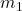= 30 kg

mass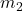= 50 kg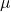= 0.1

From the question; we can arrive at two cases;

That :—– equation (1)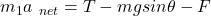—- equation (2)

50 a = 50 g – T

30 a = T – 30 g sin 30 – 4 × 30 g cos 30

By summation

80 a =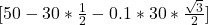g

80 a = 32. 4 × 10 m/s ²  (using g as 10m/s²)

80 a = 324 m/s ²

a = 324/80

a = 4.05 m/s²

From equation , replace a with 4.05

50 × 4.05 = 50 × 10 – T

T = 500 -202.5

T =297.5 N